0514-84242547# 关于高杆灯风载荷的设计与计算

(1) 永久载荷: 结构自重、固定的设备重、物料重、土重、土压力、线的拉力等。

(2) 可变载荷: 风载荷、裹冰载荷、常见地震作用、雪载荷、安装检修载荷、塔楼楼面或平台的活载荷、温度变化、地基沉陷等。

(3) 偶然载荷: 导线断裂、索线断线，撞击、爆炸、罕遇地震作用等。

S = μ r S o

(1) 静力理论。该理论不考虑建筑物的动力特性，假设结构物为绝对刚性，地震时建筑物运动与地面运动绝对一致，建筑物的最大加速度等于地面运动的最大加速度，建筑物所受的最大载荷等于其质量与地面最大加速度的乘积。该理论只适用于低矮的，刚性较大的建筑物。

(2) 反应谱理论。它既考虑了地震时地面的动力特性，也考虑了结构自身的动力特性，是当前工程设计应用最为广泛的地震设计方法之一。反应谱理论是以单质点体系在实际地震作用下的反应为基础来分析结构反应的方法。抗震设计中通常只需地震作用下的最大载荷值，其值为:F = ma max =w( )ga max 式中，w 为质点质量，称为水平地震影响因素。

(3) 直接动力分析理论。反应谱在分析大跨度的柔性结构时，由于非线性因素的影响，反应谱方法的计算误差较大。直接动力分析理论可以克服反应谱理论的缺点。可直接获得地震过程中结构节点各时刻位移，速度，加速度，从而计算各时刻竖向地震作用和构件的地震内力，这些理论称为直接动力分析理论。

1 设计要求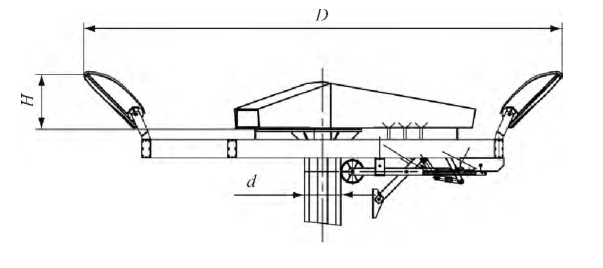2 高杆灯风载荷计算ω h= βz μ s μ z μ r ω o

(1)式中，ω h 为用在高杆灯单位面积上的风载荷 (单位: kN/m 2); ω o 为基本风压 (单位:kN/m2 ); μr为重现期调整系数，对一般高耸结构可采用 1. 1，对重要高耸结构可采用 1. 2; μ z 为 z 高度处的风压高度变化系数; μ s 为风载荷体型系数，对高杆灯选取 0. 7; β z 为 z 高度处的风振系数。

2. 1 基本风压 ω o

(2)式中，V 0 为设计风速 (单位: m/s)。将已知风速 40 米/秒代入上式，计算得出基本风压为 ω o =1 kN/m 2 。

2. 2 不同高度处的风压高度变化系数见表 1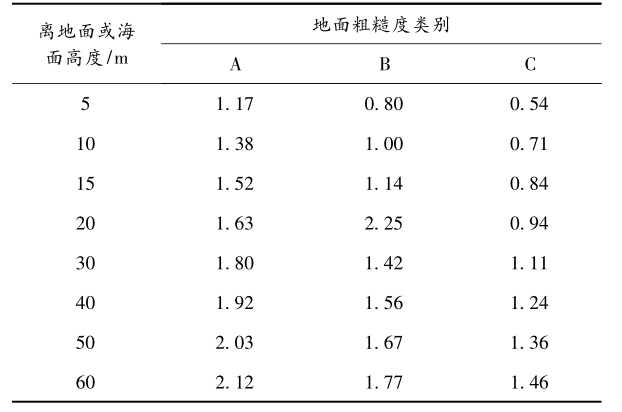2. 3 风振系数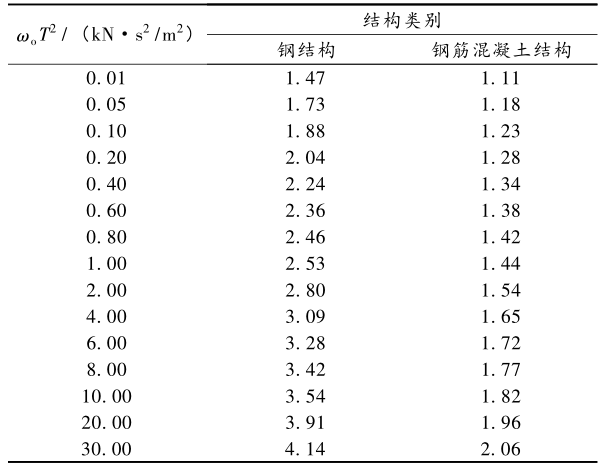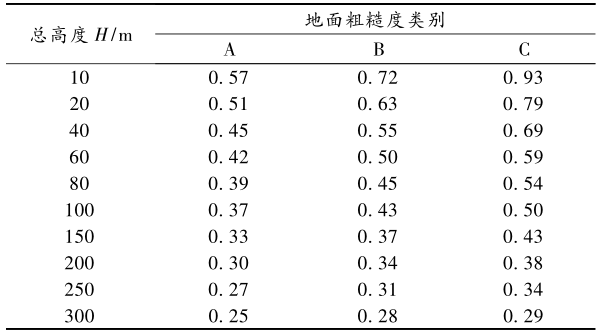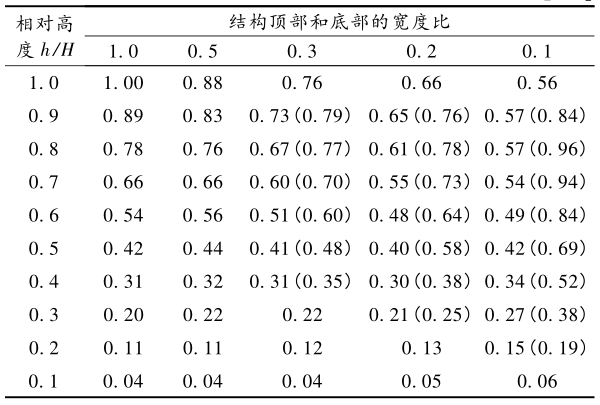β z0 =1 + ξε 1 ε 2 =1 +2. 53 ×0. 45 ×0. 88 =2

β z1 =1 + ξε 1 ε 2 =1 +2. 53 ×0. 45 ×0. 76 =1. 89

β z2 =1 + ξε 1 ε 2 =1 +2. 53 ×0. 45 ×0. 56 =1. 64

β z3 =1 + ξε 1 ε 2 =1 +2. 53 ×0. 45 ×0. 32 =1. 36

β z4 =1 + ξε 1 ε 2 =1 +2. 53 ×0. 45 ×0. 04 =1. 05

2. 4 各质量单元的单位面积风载荷

ω h0= βz0 μ s μ z0 μ r ω o =2 ×0. 7 ×1. 92 ×1. 2 ×1=3. 23 (kN/m 2 )

ω h1= βz1 μ s μ z1 μ r ω o =1. 89 ×0. 7 ×1. 80 ×1. 2 ×1=2. 86 (kN/m 2 )

ω h2= βz2 μ s μ z2 μ r ω o =1. 64 ×0. 7 ×1. 63 ×1. 2 ×1=2. 25 (kN/m 2 )

ω h3= βz3 μ s μ z3 μ r ω o =1. 36 ×0. 7 ×1. 52 ×1. 2 ×1=1. 74 (kN/m 2 )

ω h4= βz4 μ s μ z4 μ r ω o =1. 05 ×0. 7 ×1. 17 ×1. 2 ×1=1. 03 (kN/m 2 )

2. 5 各质量单元的风载荷作用力 F

(4)式中，S i 为迎风面积。

F 0= ω

h0 × S 0 =3. 23 ×4. 17 ×0. 473 ×0. 8=6. 37 (kN)

F 1= ωh1 × S 1 =2. 86 ×10. 222 =11. 14 (kN)

F 2= ωh2 × S 2 =2. 25 ×9. 753 =10. 81 (kN)

F 3= ωh3 × S 3 =1. 74 ×8. 59 =8. 86 (kN)

F 4= ωh4 × S 4 =1. 03 ×8. 435 =6. 03 (kN)

2. 6 高杆灯各段根部所受的弯矩 M

M 1 = F 0 (0. 473 +10. 222) + F 1 ( 10. 2222)=68. 11 +56. 94 =125. 05 (kNm)

M 2 = F 0 (0. 473 +10. 222 +10. 5 －0. 747) + F 1( 10. 2222+10. 5 －0. 747) + F 210. 52=130.25 +165.58 +56.75 =352.58 (kNm)

M 3 = F 0 (40. 473 －9. 5+1. 065) + F 1 (31. 889 －9. 5 +1. 065)+ F 2 (13. 185 －9. 5 +1. 065) + F 39. 5( )2=204. 08 +261. 27 +51. 34 +42. 09=558. 78 (kNm)

M 4 = F 0 (40. 473) + F 1 (31. 889)+ F 2 (22. 275) + F 3 (13. 185)+ F 4 (4. 75) =257. 81 +355. 24 +240. 79+116. 82 +28. 64 =999. 3 (kNm)

2. 7 灯杆各段根部处的应力计算

W g= π(D 4 － d 4 )32D

W g1= π(0. 442 4 －0. 430 4 )32 ×0. 442=0. 88 ×10－3(m 3 )

F g1 =M 1W g1=125. 05 ×10 30. 88 ×10－3=142. 1 ×10 6 (N/m 2 )

W g2= π(0. 543 4 －0. 527 4 )32 ×0. 543=1. 77 ×10－3(m 3 )

F g2 =M 2

W g2=352. 58 ×10 31. 77 ×10－3=199. 2 ×10 6 (N/m 2 )

W g3= π(0. 642 4 －0. 626 4 )32 ×0. 642=2. 49 ×10－3(m 3 )

F g3 =M 3

W g3=558. 78 ×10 32. 49 ×10－3=224. 4 ×10 6 (N/m 2 )

W g3= π(0. 745 4 －0. 725 4 )32 ×0. 745=4. 19 ×10－3(m 3 )

F g4 =M 4W g4=999. 3 ×10 34. 19 ×10－3=238. 5 ×10 6 (N/m 2 )

3 结束语